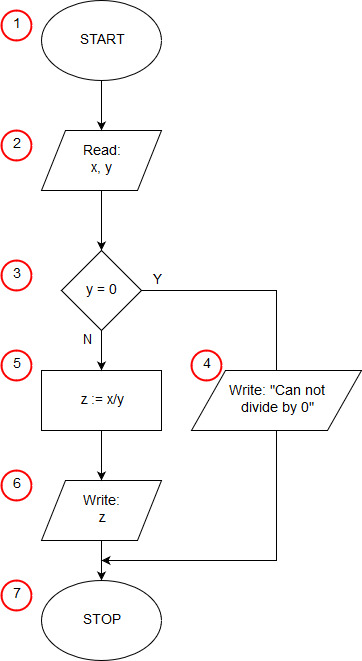# Algorithm of division in JavaScript

<<algorithm```<!DOCTYPE html>
<html>
<body>
<script>
var x=prompt("x:","");
var y=prompt("y:","");
if (y==0) alert("Can not divide by 0");
else {
var z= x/y;
};
</script>
</body>
</html>```

In 1 line, we inform the browser that our document was written in HTML (more precisely it is a header characteristic for HTML 5). Line 2 contains a <html> tag that starts HTML code. The 3 line starts the <body> section of our document, containing the elements that are to appear on the page. In our example, the <body> section only contains a script written in JavaScript that implements the algorithm for dividing two numbers. The start of the script is indicated by the <script> tag from line 4 - we can treat it as the start block (1) of our algorithm. The content of the script is contained on lines 5 to 11.

On line 5, we declare the variable x - dividend with the var keyword. at the same time, the assignment operator ("=") initializes this variable with the value entered by the user using the prompt() statement. prompt - a standard JavaScript function - displays a pop-up text box on the screen. The first argument of the function specifies the text displayed as a prompt, the second is the default value entered in the edit field. In our case, we display the text "x:" as a prompt and do not prompt the user with any default text (the second argument is empty text ""). The prompt() function returns the text entered by the user in the edit field and then confirmed with the OK button.

In line 6, we assign the divisor value to the variable y in an analogous way. Lines 5 and 6 correspond to the input/output block of this algorithm.

The conditional instruction from line 7 is the selection block (3) from our algorithm. The instruction checks if y is equal to 0. When the condition is met, the alert() instruction is executed, immediately after the condition (input/output block (4)). When the condition is not met, the instruction after the else keyword is executed, in our case it is a compound instruction enclosed in brackets {} between lines 8 and 11. First on line 9 we declare the variable z, to which we assign the value of the quotient x by y (operating block (5)), and then on line 10 we write the result (input/output block (6)).

The </script> tag on line 12 terminates the program (algorithm end block (7)).

Then on lines 13 and 14 we close the </body> and </html> sections.